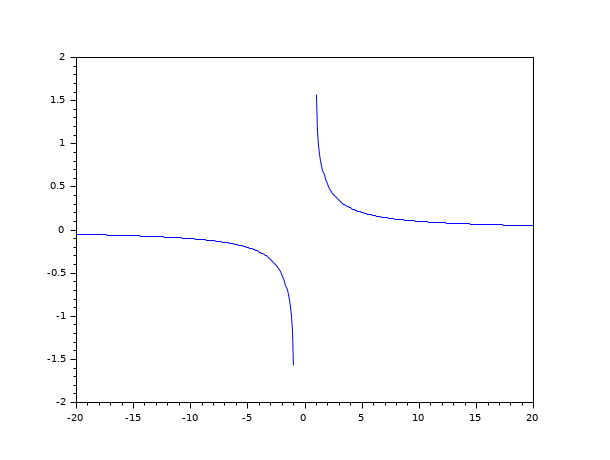Scilab Home page | Wiki | Bug tracker | Forge | Mailing list archives | ATOMS | File exchange
Change language to: Français - Português - 日本語 - Русский

# acsc

computes the element-wise inverse cosecant of the argument.

### Syntax

`y = acsc(x)`

### Arguments

x

a real or complex array.

y

a real or complex array.

### Description

Computes the element-wise inverse cosecant of the argument. For real argument with absolute value greater than 1 the result is real.

The following equalities hold: ```acsc(z) == -acsc(-z) == asin(1/z) == %pi/2-asec(z) == %i*acsch(%i*z)```

### Sample### Examples

```x=linspace(1,20,200);
x=[-x(\$:-1:1) %nan x];
plot(x,acsc(x))```

• csc — сomputes the element-wise cosecant of the argument
• acscd — computes the element-wise inverse cosecant of the argument, results in degree.
• acsch — computes the element-wise inverse hyperbolic cosecant of the argument.

### References

• Kahan, W., "Branch cuts for complex elementary functions, or, Much ado about nothing's sign bit", Proceedings of the joing IMA/SIAM conference on The State of the Art in Numerical Analysis, University of Birmingham, A. Iserles and M.J.D. Powell, eds, Clarendon Press, Oxford, 1987, 165-210.